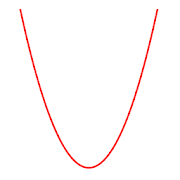# Parabola Equation Calculator - Lumos Educational App Store41
Price -fREE
\$0

#### DESCRIPTION:

Easily determine equation of a parabola! - Calculate coefficients of parabolic equation given 3 points - Save yourself time and effort - Clear results - Recalculate any number of times - Offline support

#### OVERVIEW:

Parabola Equation Calculator is a free educational mobile app By .It helps students in grades HS practice the following standards HSG.GPE.A.2.

This page not only allows students and teachers download Parabola Equation Calculator but also find engaging Sample Questions, Videos, Pins, Worksheets, Books related to the following topics.

1. HSG.GPE.A.2 : Derive the equation of a parabola given a focus and directrix.

HS

HSG.GPE.A.2

#### ADDITIONAL INFORMATION:

Developer:

Software Version: 1

Category:

### RELATED APPSEdSearch WebSearch